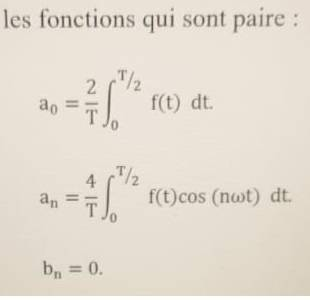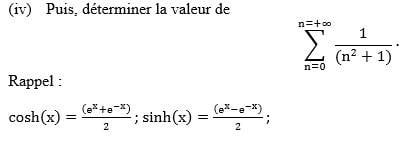# Fourier Series involving Hyperbolic Functions

• MHB
• Sharya19
In summary, Sharya19 is struggling with a math assignment that is going to be counted as a final assessment. She needs help with understanding the complex form of the Fourier series, and integrating by parts.f

#### Sharya19

Hello everyone first time here. don't know if it's the correct group... Am having some issues wiz my maths homework that going to count as a final assessment. Really Really need help.
The function (f), with a period of 2π is : f(x) = cosh(x-2π) if x [π;3π]..
I had to do a graph as the first part for the interval of [-π;5π] so that part is ok. PLEASE HELP WITH THE OTHER ISSUES...
NOTE: cosh x =(e^x + e^-x)/2

1st - Have to find out if the function is an odd or even parity... i worked it out and my answer is an even parity... But since i like to cross check(before moving on the next part) on the online calculator, it says that the function is neither odd nor even... Can someone pleasez help and then tell me How to proceed for the Fourier complex form of the function (f) if the answer is neither odd or even?

2nd - Have to find out about the Fourier complex form of the function (f).. Since i found it even parity so i used those function as seen in the picture. For ao i already did it, for the an please help wiz the integration by parts... The T in the formula i replaced by 2pi... Is it the correct way to do it?3rd - The Part Four in Roman Numeral(iv), that a total black out for mePLZ PLZ PLZ really need help...

Hello everyone first time here. don't know if it's the correct group... Am having some issues wiz my maths homework that going to count as a final assessment. Really Really need help.
The function (f), with a period of 2π is : f(x) = cosh(x-2π) if x [π;3π]..
I had to do a graph as the first part for the interval of [-π;5π] so that part is ok. PLEASE HELP WITH THE OTHER ISSUES...
NOTE: cosh x =(e^x + e^-x)/2

1st - Have to find out if the function is an odd or even parity... i worked it out and my answer is an even parity... But since i like to cross check(before moving on the next part) on the online calculator, it says that the function is neither odd nor even... Can someone pleasez help and then tell me How to proceed for the Fourier complex form of the function (f) if the answer is neither odd or even?

2nd - Have to find out about the Fourier complex form of the function (f).. Since i found it even parity so i used those function as seen in the picture. For ao i already did it, for the an please help wiz the integration by parts... The T in the formula i replaced by 2pi... Is it the correct way to do it?
View attachment 11183

3rd - The Part Four in Roman Numeral(iv), that a total black out for me
View attachment 11182
PLZ PLZ PLZ really need help...
Hi Sharya19 and welcome to MHB! Since this assignment is for assessment, we ought not to give you too much help. But here are a few hints.

I agree with you that the function is even. (The online calculator may possibly not be able to handle the way that the function is defined as being periodic with period $2\pi$, with the initial period being the interval $[\pi,3\pi]$.)

The question asks for the complex form of the Fourier series, but you have given the formula for the real Fourier series. Assuming that you want to work with the real Fourier series, you will need to find integrals of the form $$\int_0^\pi \cosh x\cos(nx)\,dx.$$ To evaluate that you need to integrate by parts twice, as follows: \begin{aligned}\int_0^\pi \cosh x\cos(nx)\,dx &= \Bigl[\sinh x\cos(nx)\Bigr]_0^\pi + n\!\!\int_0^\pi \sinh x\sin(nx)\,dx \\ &= (-1)^n\sinh\pi + n\Bigl[\cosh x\sin(nx)\Bigr]_0^\pi - n^2\!\!\!\int_0^\pi \cosh x\cos(nx)\,dx.\end{aligned} It follows that $$(1 + n^2)\!\!\int_0^\pi \cosh x\cos(nx)\,dx = (-1)^n\sinh\pi.$$ If you then use the fact that the function is equal to the sum of its Fourier series, you can evaluate the function at a suitable point (say at $x = \pi$) and you will get a formula that involves $\displaystyle \sum_{n=0}^\infty \frac1{n^2+1}.$

To evaluate that you need to integrate by parts twice, as follows:

Hello. Thanks for the clarification on the even parity. The part where it says u need to integrate by part... sorry if it sound stupid but i still can't grasp it. do i integrate cosh(x) or cosh(x-2pi)... This part is so tough to understand... pleasez help...

Hello. Thanks for the clarification on the even parity. The part where it says u need to integrate by part... sorry if it sound stupid but i still can't grasp it. do i integrate cosh(x) or cosh(x-2pi)... This part is so tough to understand... pleasez help...
The function has period $2\pi$, which means that $f(x-2\pi) = f(x)$. Also, the function takes the same values on the interval $[-\pi,\pi]$ as it does on the interval $[\pi,3\pi]$. To find the Fourier series, it is easiest to base the calculation on the fact that $f$ satisfies $f(x) = \cosh x$ on the interval $[-\pi,\pi]$.

The function has period $2\pi$, which means that $f(x-2\pi) = f(x)$. Also, the function takes the same values on the interval $[-\pi,\pi]$ as it does on the interval $[\pi,3\pi]$. To find the Fourier series, it is easiest to base the calculation on the fact that $f$ satisfies $f(x) = \cosh x$ on the interval $[-\pi,\pi]$.
Sorry if the question sound stupid again... My limit is pi to zero?

Sorry if the question sound stupid again... My limit is pi to zero?
Yes, the function is even, so you can take twice the integral over the interval $[0,\pi]$ to get the integral over $[-\pi,\pi]$.

Yes, the function is even, so you can take twice the integral over the interval $[0,\pi]$ to get the integral over $[-\pi,\pi]$.
Thanks a lot dr

Yes, the function is even, so you can take twice the integral over the interval $[0,\pi]$ to get the integral over $[-\pi,\pi]$.
Hello. sorry to disturb again. so if i have to find the complex form of the Fourier serie for the function (f) above.
The equation will be cn=1/2pi integral (pi to -pi) f(x) e^inx dx?? Please confirm..

Hello. sorry to disturb again. so if i have to find the complex form of the Fourier serie for the function (f) above.
The equation will be cn=1/2pi integral (pi to -pi) f(x) e^inx dx?? Please confirm..
Yes. You will find that it is much easier to calculate the complex Fourier coefficients than the real ones. It is no longer necessary to use integration by parts.

Yes. You will find that it is much easier to calculate the complex Fourier coefficients than the real ones. It is no longer necessary to use integration by parts.
Thanks a lot dr. God bless u